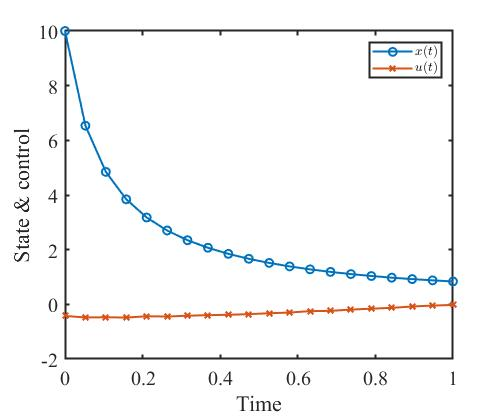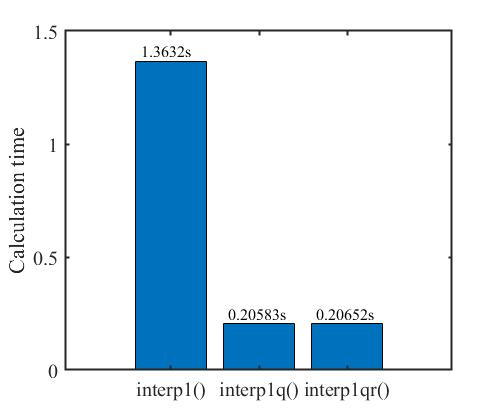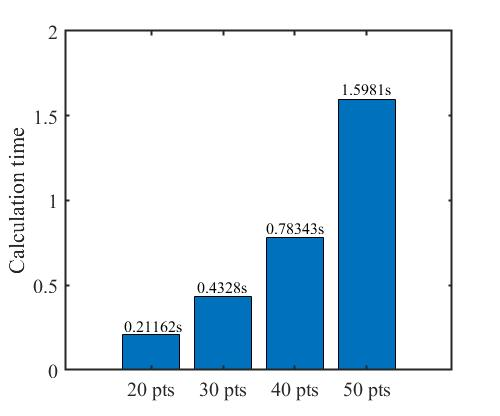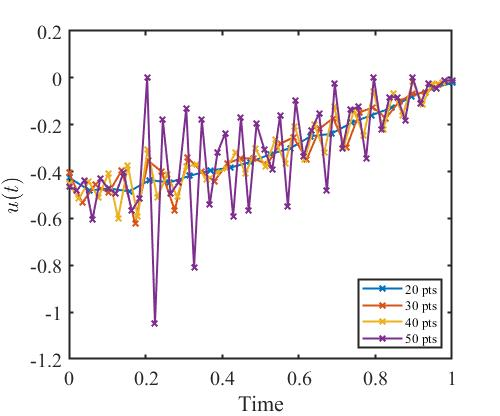# 写在前面

• 打靶法，特点为仅离散控制变量；
• 配点法，特点为同时离散状态变量和控制变量。

# 打靶法

\begin{aligned} t_0 &= t_1 < t_2 < \dots < t_N = t_f, \\ \boldsymbol{y} &= (\boldsymbol{u}_1, \boldsymbol{u}_2, \dots, \boldsymbol{u}_N), \\ u_k(t) &= \varphi_u(t, u_{k1}, u_{k2}, \dots, u_kp) \quad k = 1, 2, \dots, m, \\ u_k(t) &= u_{k(i)} + \frac{u_{k(i)}-u_{k(i-1)}}{t_i-t_{i-1}}(t-t_{i-1} \quad t_{i-1} \le t \le t_i). \end{aligned}

• 性能指标；
• 控制系统微分方程约束；
• 边界约束；
• 路径约束。

## 性能指标转化过程

$J = \Phi (\mathbf{x}(t_0),t_0,\mathbf{x}(t_f),t_f) + \int_{t_0}^{t_f} L(\mathbf{x}(t),\mathbf{u}(t),d) \text{d}t$

$\Phi (\mathbf{x}(t_0),t_0,\mathbf{x}(t_f),t_f),$

$JB (u(t),t_0,t_f),$

$\int_{t_0}^{t_f} L(\mathbf{x}(t),\mathbf{u}(t),d) \text{d}t$

$JL(u(t),t),$

$JB (u(t),t_0,t_f) + JL(u(t),t).$

## 控制系统微分方程约束转化过程

$\boldsymbol{\dot x}(t) = \boldsymbol{f}(\boldsymbol{x}(t),\boldsymbol{u}(t),t).$

$\boldsymbol{x}(t) = g(u(t),t),$

## 边界约束转化过程

$\phi (\boldsymbol{x}(t_0),t_0,\boldsymbol{x}(t_f),t_f) = 0.$

$CB(u(t),t_0,t_f) = 0.$

## 路径约束转化过程

$\mathbf{C}(\mathbf{x}(t),\mathbf{u}(t),t) \le 0.$

$CP(u(t),t) = 0.$

# 算法步骤

1. 确定最优控制问题的离散点数、起止时间、状态变量初值、控制变量初值；
2. 计算控制变量数量和约束条件数量：
• 控制变量数量 = 控制变量维度 * 离散点数，
• 约束条件数量 = 状态变量维度 * 离散点数 + 边界条件数量 + 路径约束数量 * 离散点数，
• 若终端时间不固定，则需将设计变量个数加 1。
3. 将目标函数转化为离散形式；
4. 调用 matlab 的 fmincon() 求解该 NLP 问题。

# 代码

%--------------------------------------------------------------------------
% 说明：应用直接打靶法求解无约束最优控制问题
% 例子：《最优化与最优控制》 pp. 257 例13.1
% 类型：无控制约束的最优控制问题
% 时间：2022/07/01
%--------------------------------------------------------------------------
clear;clc;close all;

%% 01 初始参数设置
p.ns = 1; p.nu = 1;                     % 状态量个数和控制量个数
p.t0 = 0; p.tf = 1;                     % 初始时间和终止时间
p.x0 = 10;                              % 初始条件

% 直接打靶法参数设置
p.nt = 20;                              % 打靶点参数设置
p.t = linspace(p.t0,p.tf,p.nt)';        % 时间区间

% 将控制量离散
p.u_index = 1:p.nt;

%% 02 求解算法
% 给控制量赋初值
p.u = -0.5*ones(p.nt,1);
u0 = p.u;              % 控制量的初值猜测

% 求解控制量
options = optimoptions(@fmincon,'display','iter','MaxFunEvals',1e5,'algorithm','sqp');
tic;
[u,fval,exitflag,output] = fmincon(@(u) objective(u,p),u0,[],[],[],[],[],[],[],options);
toc;
p.u = u;
time_record = toc;

% 再进行一次仿真得到数据
[~,Y] = ode45(@(t,y) derivative(t,y,p),p.t,p.x0);
p.x = Y(:,1);

%% 画图
k = 0;
window_width = 500;
window_height = 416;

% 状态量
fig.k = 0;
figure('color',[1 1 1],'position',[300+k*window_width,300,window_width,window_height]);
plot(p.t, p.x, 'x-', 'LineWidth',1.5);hold on;
plot(p.t, p.u, 'x-', 'LineWidth',1.5);
xlabel('Time');
ylabel('State & control');
set(gca,'FontSize',15,...
'FontName','Times New Roman',...
'LineWidth',1.5);
legend('$x(t)$','$u(t)$',...
'Location','Northeast',...
'FontSize',10,...
'interpreter','latex');

% 保存数据
% .\ 下一级文件夹
% ..\ 上一级文件夹
% save(['..\','shooting_method.mat']);

%% 子函数部分
% 目标函数
function f = objective(u_obj,p)
p.u = u_obj(p.u_index);
[~,Y] = ode45(@(t,y) derivative(t,y,p),p.t,p.x0); % 仿真得到时序状态量
x = Y;                          % 状态量
u = u_obj(p.u_index);           % 控制量
L = u.^2/2 + x.^2/2;            % 积分项
f = trapz(p.t,L);               % 计算目标函数
end

% 状态方程
function dy = derivative(t,y,p)
% 使用 interp1qr() 进行插值
%     u = interp1qr(p.t,p.u,t);
% 使用 interp1q() 函数进行插值
% 发现 interp1q() 的速度比 interp1() 快，和nterp1qr() 速度一样
u = interp1q(p.t,p.u,t);
% 使用 interp1() 函数进行插值
%     u = interp1(p.t,p.u,t);
dy = -y^2 + u;
end


# 结果# 对比

## 不同插值函数对计算时长的影响

• 处理器：Intel(R) Core(TM) i5-8265U CPU @ 1.60GHz x86
• 内存： DD4R 2400 MHz 8GB + DD4R 2667 MHz 8GB
• 电源模式：平衡模式

matlab中有个 interp1q() 函数，但目前 mathwork 已不推荐使用该函数。不过用它进行插值的速度十分快，这个函数是一种快速一维插值方法，运行速度比 interp1() 更快，但因为提供的插值方式少，且代码编写年代久远，所以已经不推荐使用该函数。不过在本算例中还是可以继续发挥作用的。

interp1(Xvec,Vvec,xq,method) 对于 Xvecxq 中的插值处理，是将 Xvec 从前至后排列好，再依次比较大小，最后定位到特定的时间区段内。

for i = 1:length(Xvec) - 1
if xq < Xvec(i) && xq > Xvec(i+1)
在 [Xvec(i), Xvec(i+1)] 区间内线性插值
else
继续比较大小，定位 xq 的位置，确定使用哪一段的 [Xvec(i), Xvec(i+1)] 区间
end
end


It runs at least 3x faster than ‘interp1q’ and 8x faster than ‘interp1’, and more than 10x faster as m=length(x) increases (see attached performance graph).interp1q()interp1qr() 的计算时长基本一致，没有如同作者说的 It runs at least 3x faster than ‘interp1q’ ，原因或许是算例问题比较简单，没有发挥出来 interp1qr() 的优势，在后面的学习过程中说不定会有新的体会和发现。interp1() 的计算时长最长，因为它在使用过程中进行了很多检查工作，但这些检查工作其实没有必要，写代码的时候已经检查过一遍了，出错几率低。调用次数少的时候，检查一下没有关系，但调用次数多了之后，每回都要检查，这无疑加重了计算机的负担；另外，interp1() 的插值方式存在缺陷，这又是导致计算时间长的原因。

## 不同离散点数对计算时长的影响1. 关于更新步长的选择问题，孙涛于2021年发表在《运筹学学报》的论文《梯度法简述》里讨论了4种不同的梯度法步长更新策略。

2. K. L. Teo, C. Goh, et al. A unified computational approach to optimal control problems. 1991.